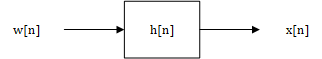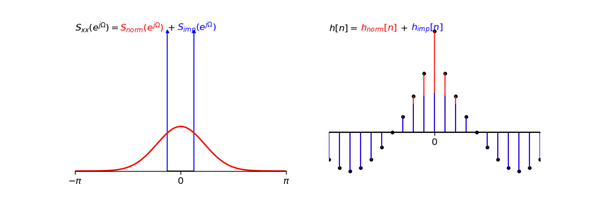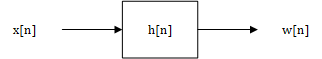2018/06/27 23:55

# Colored and White Process

#### White Process

White Process，又称为White Noise（白噪声），其中white来源于白光，寓意着PSD的平坦分布，white noise指的就是在PSD上具有平坦分布（常数）的随机过程。PSD是auto-correlation的傅里叶变换，PSD上为常数意味着auto-correlation是一个位于零点上的脉冲函数。

$R_{xx}(\tau) = E\Big\{x(t+\tau)x(t)\Big\}$

Auto-correlation在零点上为脉冲，除此之外都是0，这表明了各个采样点之间是相互独立的，正是由于各采样点之间相互独立，才体现出了white noise的white这一特点

i.i.d. process就是一个最简单white noise的例子。i.i.d. process中样本的PDF常见的有Uniform、Poisson、Gaussian等分布方式，如果PDF为Gaussian，则该process称为Gaussian white noise。

#### Colored Process

PSD表现得平坦的就是white noise，否则就是colored process。

# Modeling Filter

$\displaystyle{ H(e^{j\Omega}) = \sqrt{S_{xx}(e^{j\Omega})} }$• 脉冲在零点。那么脉冲PSD的脉冲响应是一个常数，该常数的大小与脉冲PSD的大小有关。
• 脉冲在$\Omega = \pm \Omega_0\neq 0$上。那么脉冲PSD的脉冲响应是一个余弦函数$Acos(\Omega_0 n+\Theta)$，其中$A$以及$\Theta$都与脉冲PSD的大小有关。※这一段只是计算时需要，不算理解时的重点，如想加深了解，请参考常用序列的DTFT

# Whitening Filter$\displaystyle{S_{ww}(e^{j\Omega}) = |H(e^{j\Omega})|^2S_{xx}(e^{j\Omega})}$

$\displaystyle{|H(e^{j\Omega})|^2 = \frac{\sigma_w^2}{S_{xx}(e^{j\Omega})}}$

Reference:

Alan V. Oppenheim: Signals, Systems and Inference, Chapter 10:Power Spectral Density

Where does the delta function come from if we derive autocorrelation directly?

0
0 收藏

### 作者的其它热门文章0 评论
0 收藏
0